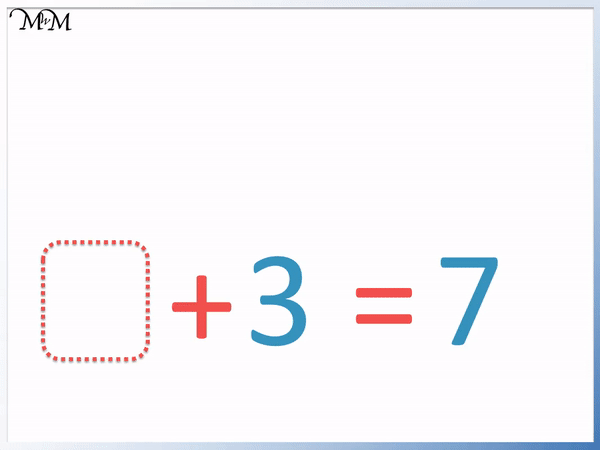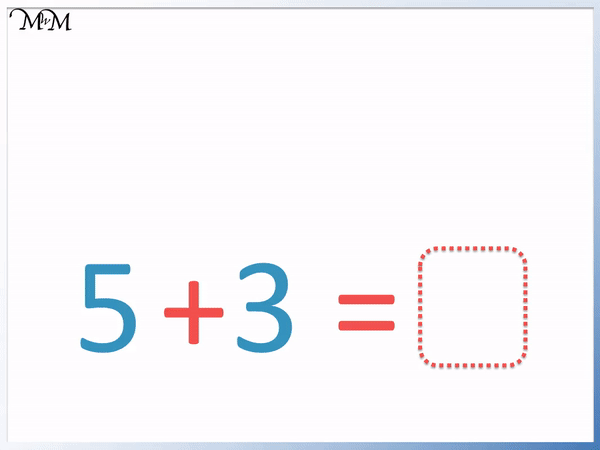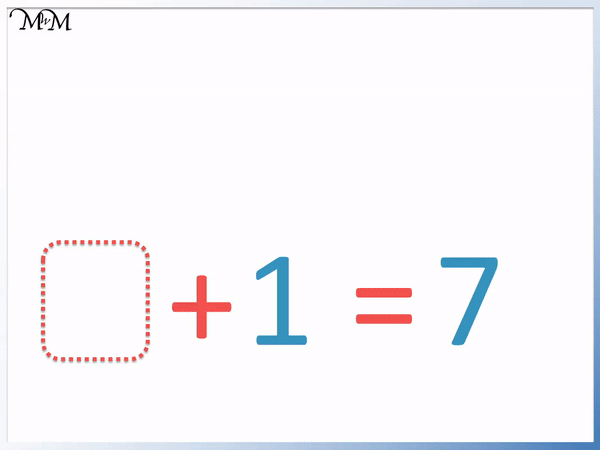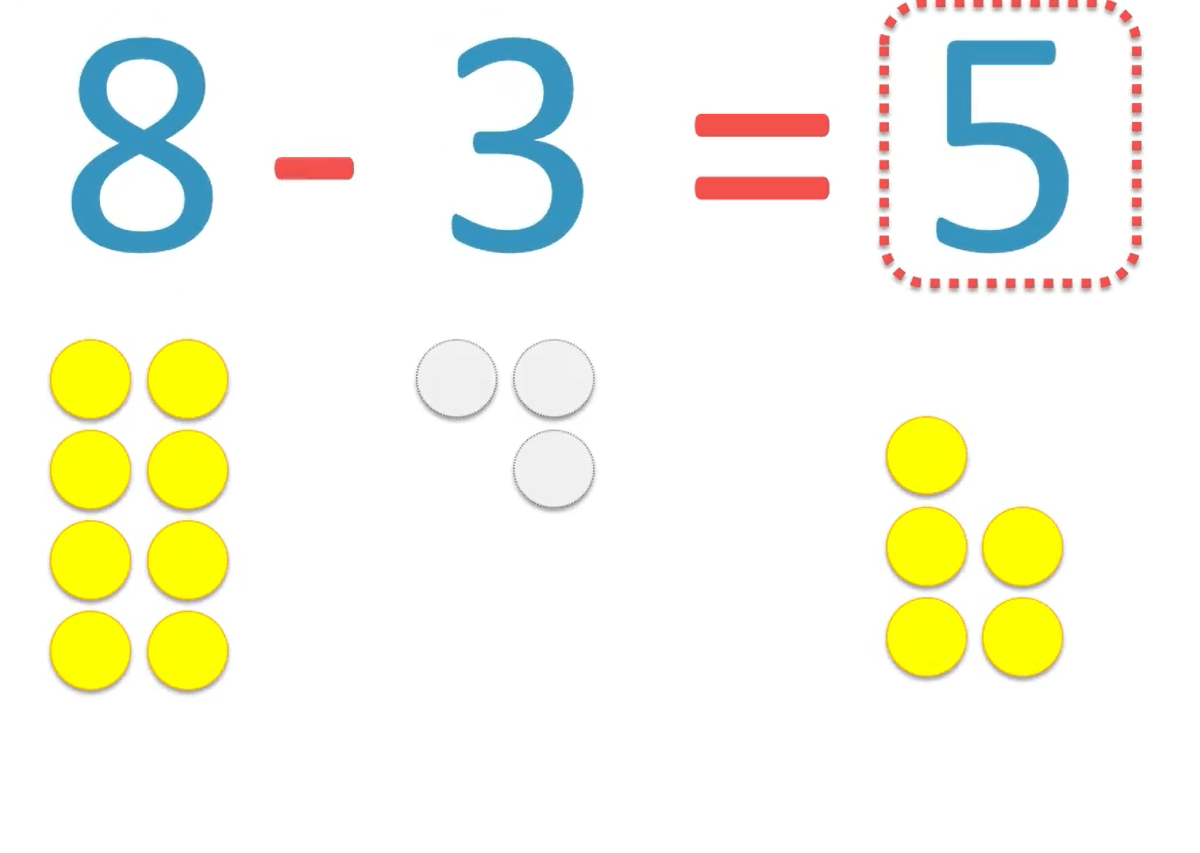• Here is an addition sentence with a missing number at the end.
• This missing number is equal to 5 + 3.
• The number must be the same value as 5 and 3 combined.
• Five add three equals eight.
• If the missing number in the addition sentence is after the equals sign then we can just evaluate the sum before the equals sign.• Here is the same addition sentence with a different missing number.
• This sentence is telling us that 5 add the missing number will equal eight.
• We can count on from five until we make eight.
• We need to count three more from five to make eight and so, 3 is the missing number.
• We can see the similarity to the missing number problem in the previous image and use this to help us.

If the missing number is after the equals sign then we can work out the value of the sum before the equals sign.

If the missing number is added to another number we need to work out what we add to the other number to get our answer.• In this problem we have a missing number at the start of the addition sentence.
• To solve the missing number problem we think, ‘what do we add to 3 to make 7?’.
• We can start at 3 and count on: 4, 5, 6, 7, which is four more.
• We add 4 to 3 to make 7.
• We can also work this out by subtracting 3 from 7.
• 7 – 3 = 4.Supporting Lessons# How to Solve Missing Number Problems in Addition Sentences

In this lesson we are learning to solve missing number problems.

A missing number problem is a number sentence in which one or more numbers need to be filled in to complete it.

We will be learning how to find missing numbers in addition sentences, which involve a plus sign ‘+’ and an equals sign ‘=’.

We will begin with an example of the most commonly seen missing number problem, in which the missing number is at the end of the number sentence, after the equals sign.

In this first type of missing number problem, the missing number is simply the answer to the addition and it looks like a regular sum.

Here is the first example:Addition sentences all contain a plus sign ‘+’ and addition means that we combine the values of the numbers either side of the plus sign.

In this example, to find the number in the box we think:

‘what is the total of 3 add 1?’

3 + 1 = 4 and so we write the missing number 4 in the box.

If the missing number is at the end of the number sentence after the equals sign, we can simply work out the sum before the equals sign.

Here is another example like this.Since the missing number is after the equals sign, we simply need to work out the value of the sum before the equals sign.

We can add 5 + 3 by counting on three from five.

5 + 3 = 8

We can use this example to help us work out missing number problems where the missing number is due to be added to another number.

Below we have the same example but this time we have the answer of 8 and we have a missing number to be added to the five.The easiest way to solve this is to compare it to the previous question, in which we already knew that 5 + 3 = 8.

Knowing addition facts can help us solve these missing number problems most fluently. Addition facts are combinations of pairs of numbers that add up to a given number.

However we can also count on from 5 and see how many more numbers we need to add to 5 to reach 8.

After 5 there is 6, 7 and 8 which is 3 more numbers.

Here is another example of an addition sentence with a missing number before the equals sign.

We will first solve this missing number problem by counting on from 3 to see how many more numbers we need to add to reach 6.Starting at 3 and counting on, the numbers are: 4, 5 and 6.

This is 3 more numbers and so we write the missing number as 3.

When teaching these missing number problems, this counting on method can help to support the addition, however the aim is to build the knowledge of number facts first to make this process easier.

Knowing the addition fact that 3 + 3 = 6 means that the missing number can be simply written in.

To help remember the addition facts such as this, you can practise working them out as you encounter them. To work out that the missing number is 3, we can simply subtract the other number from 6.

6 – 3 = 3

We can just subtract the other number that we are adding from the answer.

Here is another example of finding a missing number in this position.

To solve this problem we think:

‘what number do we add to 7 to make 9?’If we know our addition facts then we know that:

7 + 2 = 9

Alternatively we can subtract the other given number away from the total.

9 – 7 = 2

and so, we write the missing number as 2.

In this example, the missing number is at the beginning of the number sentence.The missing number will be the number that we add to 1 to make the total of 7.

We can start at 1 and count on until we reach 7.

Alternatively, we know the addition fact that 6 + 1 = 7 and hence the missing number in the box in 6.

The missing number is still part of the addition as it is directly next to the plus sign ‘+’.

So, to find the missing number we can subtract the other number next to the plus sign away from the total.

7 – 1 = 6

6 is the missing number that we write in the box.

Here is our last example of finding a missing number in an addition.Again the missing number is at the start of the number sentence.

It is not so much, where the missing number is in the equation but what it is next to.

It is next to the addition sign ‘+’ and so we know that we are combining its value with the other number, 3.

We think ‘what do we add to 3 to make 7?’.

We can count on from 3 until we reach the total of 7.

We have: 3 and then 4, 5, 6, 7, which is four more numbers.

Alternatively, we know the addition fact that 4 + 3 = 7.

We write the missing number of 4 in the box.

Since our missing number is next to the plus sign, we can subtract the other number next to the plus sign away from the total.

7 – 3 = 4

When teaching finding missing numbers in addition problems, we first need to identify which kind of missing number it is.

Either the missing number is at the end of the number sentence, alone after the equals sign or it is next to the addition sign.

If the number is after the equals sign, then it will be the result of adding the other numbers in the addition sentence (provided that we have a simple addition). If the number is next to the addition sign and we are given the total then we have three main strategies:

• Count on from the other number to see how many more it is to reach the total.
• Use number facts to remember which numbers add to make the given total.
• Subtract the other number next to the addition sign away from the total.Now try our lesson on Missing Numbers in Subtraction where we learn how to find missing numbers in subtraction number sentences.error: Content is protected !!